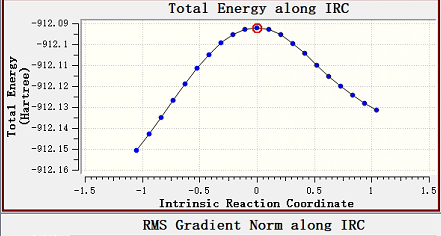# How to obtain IRC figure

But we have encountered a new problem. We would like to get the total energy along the IRC direction, but we cannot find the values of the intrinsic reaction coordinate. And through I-Qmol only GEOMETRY vs Energy figure can be observed. Waiting for your reply.And How is the IRC figure obtained?As mentioned here:

the string is not a rigorous IRC. To determine the latter, use JOBTYPE=RPATH.

Thank you for your help. We calculate the transition state from reactant and product structures and added “JOBTYPE rpath”. The input file command is as follows:
\$rem
JOBTYPE fsm
METHOD pbe0
DFT_D = D3_BJ
BASIS aug-cc-pVTZ
FSM_NNODE 18
FSM_MODE 2
FSM_OPT_MODE 2
SYMMETRY false
SYM_IGNORE true
\$end

@@@

\$molecule
\$end

\$rem
JOBTYPE ts
METHOD pbe0
DFT_D = D3_BJ
BASIS aug-cc-pVTZ
THRESH 14
SCF_ALGORITHM DIIS
SCF_CONVERGENCE 8
SCF_MAX_CYCLES 1000000000
MAX_SCF_CYCLES 250000000
MAX_DIIS_CYCLES=100000000
GEOM_OPT_DMAX 50
GEOM_OPT_MAX_CYCLES 10000000
GEOM_OPT_TOL_DISPLACEMENT=60
GEOM_OPT_TOL_ENERGY 20
mem_total = 85000
SYMMETRY false
SYM_IGNORE true
\$end

@@@

\$molecule
\$end

\$rem
JOBTYPE freq
BASIS aug-cc-pVTZ
METHOD pbe0
DFT_D = D3_BJ
\$end

@@@

\$molecule
\$end

\$rem
JOBTYPE rpath
BASIS aug-cc-pVTZ
METHOD pbe0
DFT_D = D3_BJ